• Pytorch LSTM 时间序列预测 https://github.com/pytorch/examples/blob/master/time_sequence_prediction/generate_sine_wave.py Pytorch官网提供初学者入门的一个例子，有助于学习Pytorch时间序列预测。本例中...
Pytorch  LSTM 时间序列预测

https://github.com/pytorch/examples/blob/master/time_sequence_prediction/generate_sine_wave.py

Pytorch官网提供初学者入门的一个例子，有助于学习Pytorch时间序列预测。本例中使用两个LSTMCell单元学习从不同相位开始的一些正弦波信号,LSTM网络在学习了正弦波之后，试图预测未来的信号值。

generate_sine_wave.py生成模拟数据：

# -*- coding: utf-8 -*-

import numpy as np
import torch

np.random.seed(2)

T = 20
L = 1000
N = 100

x = np.empty((N, L), 'int64')

x[:] = np.array(range(L)) + np.random.randint(-4 * T, 4 * T, N).reshape(N, 1)
data = np.sin(x / 1.0 / T).astype('float64')
torch.save(data, open('traindata.pt', 'wb'))



LSTM数据序列预测：

# -*- coding: utf-8 -*-
from __future__ import print_function
import torch
import torch.nn as nn
import torch.optim as optim
import numpy as np
import matplotlib
matplotlib.use('Agg')
import matplotlib.pyplot as plt

class Sequence(nn.Module):
def __init__(self):
super(Sequence, self).__init__()
self.lstm1 = nn.LSTMCell(1, 51)
self.lstm2 = nn.LSTMCell(51, 51)
self.linear = nn.Linear(51, 1)

def forward(self, input, future = 0):
outputs = []
h_t = torch.zeros(input.size(0), 51, dtype=torch.double)
c_t = torch.zeros(input.size(0), 51, dtype=torch.double)
h_t2 = torch.zeros(input.size(0), 51, dtype=torch.double)
c_t2 = torch.zeros(input.size(0), 51, dtype=torch.double)

for i, input_t in enumerate(input.chunk(input.size(1), dim=1)):
h_t, c_t = self.lstm1(input_t, (h_t, c_t))
h_t2, c_t2 = self.lstm2(h_t, (h_t2, c_t2))
output = self.linear(h_t2)
outputs += [output]
for i in range(future):# if we should predict the future
h_t, c_t = self.lstm1(output, (h_t, c_t))
h_t2, c_t2 = self.lstm2(h_t, (h_t2, c_t2))
output = self.linear(h_t2)
outputs += [output]
outputs = torch.stack(outputs, 1).squeeze(2)
return outputs

if __name__ == '__main__':
# set random seed to 0
np.random.seed(0)
torch.manual_seed(0)
# load data and make training set
input = torch.from_numpy(data[3:, :-1])
target = torch.from_numpy(data[3:, 1:])
test_input = torch.from_numpy(data[:3, :-1])
test_target = torch.from_numpy(data[:3, 1:])
# build the model
seq = Sequence()
seq.double()
criterion = nn.MSELoss()
# use LBFGS as optimizer since we can load the whole data to train
optimizer = optim.LBFGS(seq.parameters(), lr=0.8)
#begin to train
for i in range(15):
print('STEP: ', i)
def closure():
out = seq(input)
loss = criterion(out, target)
print('loss:', loss.item())
loss.backward()
return loss
optimizer.step(closure)
# begin to predict, no need to track gradient here
future = 1000
pred = seq(test_input, future=future)
loss = criterion(pred[:, :-future], test_target)
print('test loss:', loss.item())
y = pred.detach().numpy()
# draw the result
plt.figure(figsize=(30,10))
plt.title('Predict future values for time sequences\n(Dashlines are predicted values)', fontsize=30)
plt.xlabel('x', fontsize=20)
plt.ylabel('y', fontsize=20)
plt.xticks(fontsize=20)
plt.yticks(fontsize=20)
def draw(yi, color):
plt.plot(np.arange(input.size(1)), yi[:input.size(1)], color, linewidth = 2.0)
plt.plot(np.arange(input.size(1), input.size(1) + future), yi[input.size(1):], color + ':', linewidth = 2.0)
draw(y, 'r')
draw(y, 'g')
draw(y, 'b')
plt.savefig('predict%d.pdf'%i)
plt.close()

运行结果：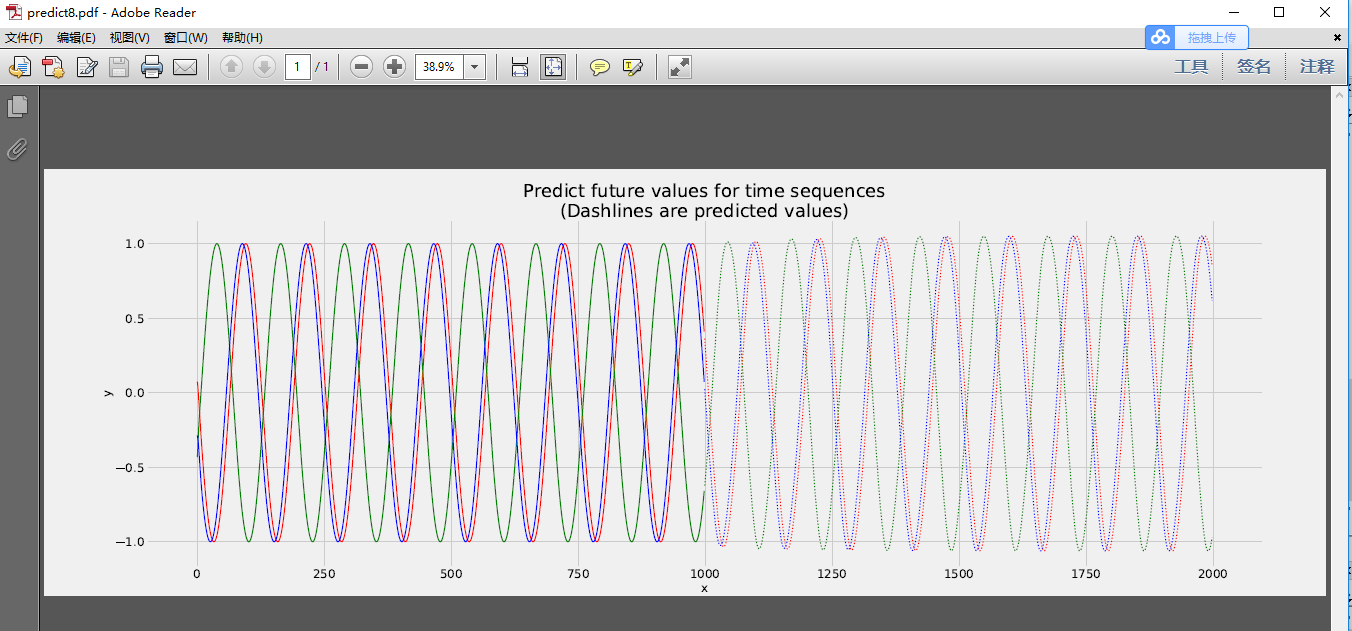展开全文• 各位朋友大家好，今天来讲一下LSTM时间序列的预测进阶。 现在我总结一下常用的LSTM时间序列预测： 1.单维单步（使用前两步预测后一步） 可以看到trainX的shape为 (5,2) trainY为(5,1) 在进行训练的过程中要将...
各位朋友大家好，今天来讲一下LSTM时间序列的预测进阶。
现在我总结一下常用的LSTM时间序列预测：

1.单维单步（使用前两步预测后一步）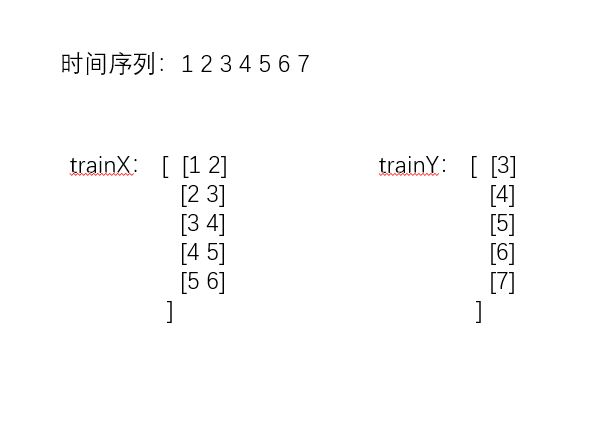可以看到trainX的shape为 (5,2)     trainY为(5,1)
在进行训练的过程中要将trainX reshape为 (5,2,1)(LSTM的输入为 [samples, timesteps, features]
这里的timesteps为步数，features为维度 这里我们的数据是1维的)
2.单维多步（使用前两步预测后两步）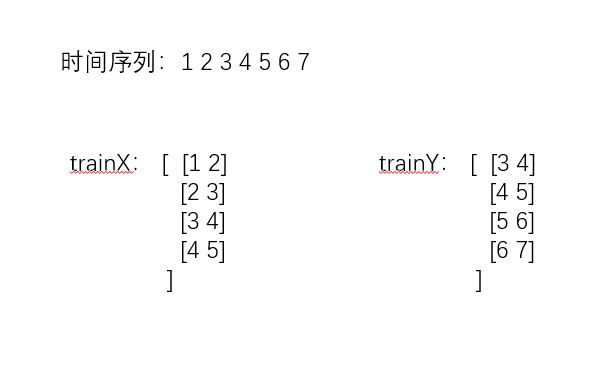可以看到trainX的shape为 (4,2)     trainY为(4,2)
同样的，在进行训练的过程中要将trainX reshape为 (5,2,1)
3.多维单步（使用前三步去预测后一步）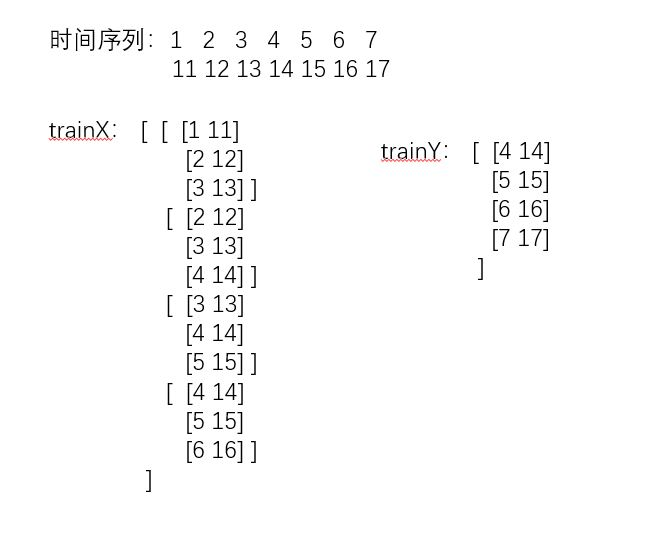可以看到trainX的shape为 (4,3,2)   [samples = 4, timesteps =3, features = 2]  trainY为(4,2)
4.那么切入正题，我们进行多维多步的预测（使用前三步去预测后两步）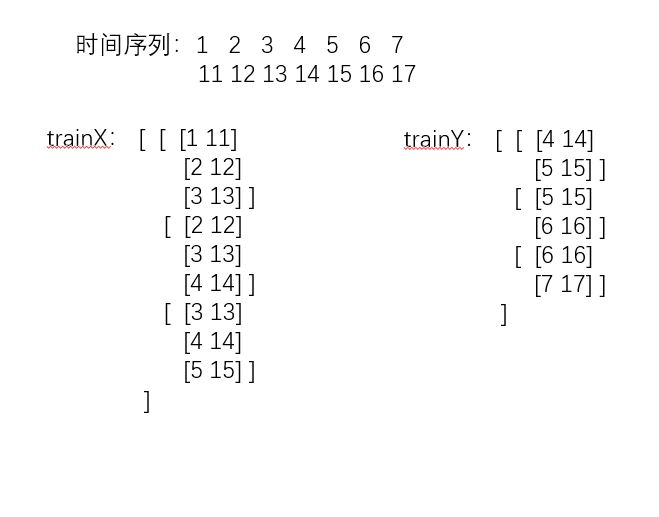可以看到trainX的shape为 (3,3,2)   trainY为(3,2,2)
那么问题来了。使用LSTM进行时间序列预测的网络结构如下：
model = Sequential()
80,
input_shape=(trainX.shape, trainX.shape),
return_sequences=True))
80,
return_sequences=False))
trainY.shape))
model.fit(trainX, trainY, epochs=50, batch_size=64 ,verbose = 2)

可以看到最后的输出是一个全连接层，也就是最终只能输出一个长度为trainY.shape的数组。
所以trainY最多只能是一个二维的矩阵，三维的trainY并不能运行。
那么我们应该怎么办呢？
把trainY由三维转为二维。当进行预测之后，把预测结果再由二维转为三维。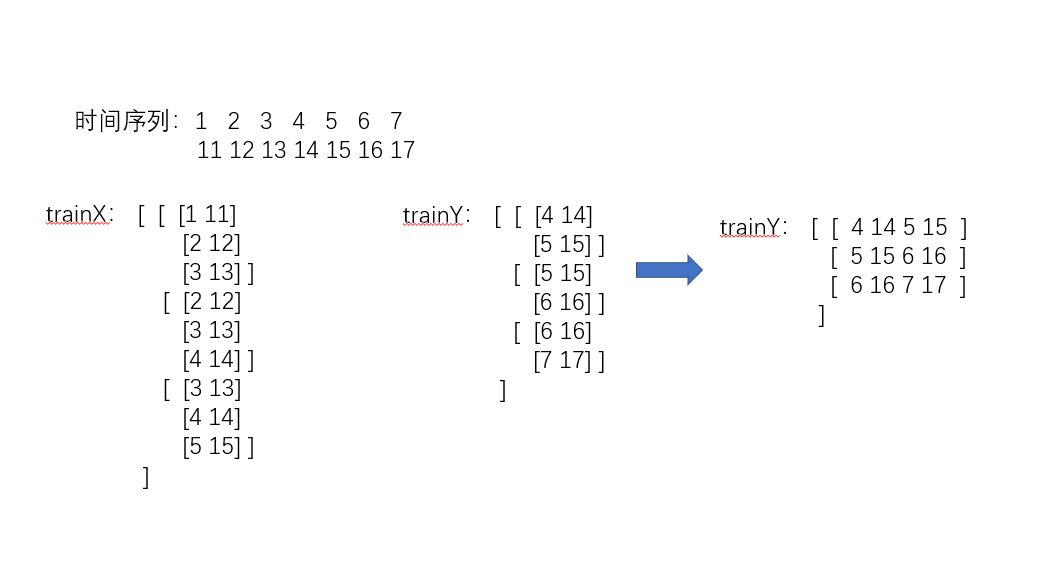此时的trainX的shape为 (3,3,2)   trainY为(3,4) 我们只要记住trainY是如何从三维转化成二维的，再将预测值（二维）按照顺序转化回去即可。
进行训练
转化函数
def create_dataset(data,n_predictions,n_next):
'''
对数据进行处理
'''
dim = data.shape
train_X, train_Y = [], []
for i in range(data.shape-n_predictions-n_next-1):
a = data[i:(i+n_predictions),:]
train_X.append(a)
tempb = data[(i+n_predictions):(i+n_predictions+n_next),:]
b = []
for j in range(len(tempb)):
for k in range(dim):
b.append(tempb[j,k])
train_Y.append(b)
train_X = np.array(train_X,dtype='float64')
train_Y = np.array(train_Y,dtype='float64')

return train_X, train_Y

模型训练函数
def trainModel(train_X, train_Y):
'''
trainX，trainY: 训练LSTM模型所需要的数据
'''
model = Sequential()
140,
input_shape=(train_X.shape, train_X.shape),
return_sequences=True))

140,
return_sequences=False))

train_Y.shape))

model.fit(train_X, train_Y, epochs=100, batch_size=64, verbose=1)

return model

我们拟定一个二维的数据对其进行实验 这个数据长度是1000条，一维是sin函数 另一维是cos函数
使用前200步去预测后50步
#进行测试
data = np.zeros(2000)
data.dtype = 'float64'
data = data.reshape(1000,2)
sinx=np.arange(0,40*np.pi,2*np.pi/50,dtype='float64')
siny=np.sin(sinx)
cosx=np.arange(0,40*np.pi,2*np.pi/50,dtype='float64')
cosy=np.cos(sinx)

data[:,0] = siny
data[:,1] = cosy

print(data)
plt.plot(data[:,0])
plt.show()
plt.plot(data[:,1])
plt.show()
#归一化的加入
data,normalize = NormalizeMult(data)

train_X,train_Y = create_dataset(data,200,50)
model = trainModel(train_X,train_Y)

np.save("./MultiSteup2.npy",normalize)
model.save("./MultiSteup2.h5")

注：这里加入了归一化，因为如果不进行归一化的话会导致loss降不下去
当训练完成后 我们使用一个shape为（1,200,2）的test_X去预测得到的y_hat是一个（1，100）的矩阵
所以我们还需要将y_hat转化为（50，2）的矩阵
转化函数为：
def reshape_y_hat(y_hat,dim):
re_y = []
i = 0
while i < len(y_hat):
tmp = []
for j in range(dim):
tmp.append(y_hat[i+j])
i = i + dim
re_y.append(tmp)
re_y = np.array(re_y,dtype='float64')
return  re_y

进行测试
#仅对最后200条数据进行测试 因为预测仅最新有作用
data = np.zeros(400)
data.dtype = 'float64'
data = data.reshape(200,2)
sinx=np.arange(0,8*np.pi,2*np.pi/50,dtype='float64')
siny=np.sin(sinx)
cosx=np.arange(0,8*np.pi,2*np.pi/50,dtype='float64')
cosy=np.cos(sinx)
data[:,0] = siny
data[:,1] = cosy

#归一化
data = NormalizeMultUseData(data, normalize)
test_X = data.reshape(1,data.shape,data.shape)
y_hat  =  model.predict(test_X)
#重组
y_hat = y_hat.reshape(y_hat.shape)
y_hat = reshape_y_hat(y_hat,2)

#反归一化
y_hat = FNormalizeMult(y_hat, normalize)

print(y_hat.shape)
plt.plot(y_hat[:,0])
plt.show()
plt.plot(y_hat[:,1])
plt.show()

得到的结果为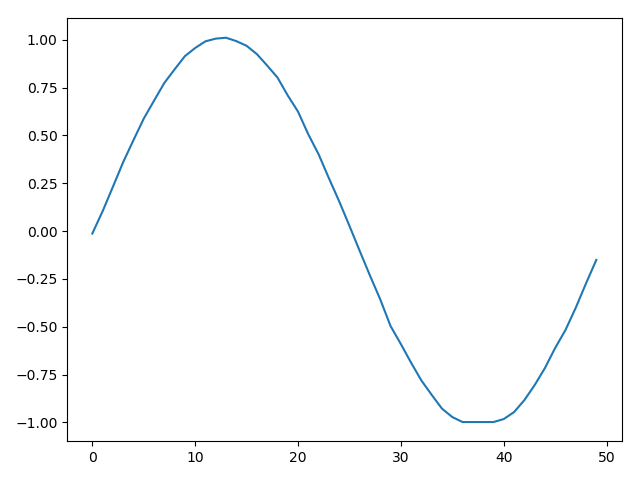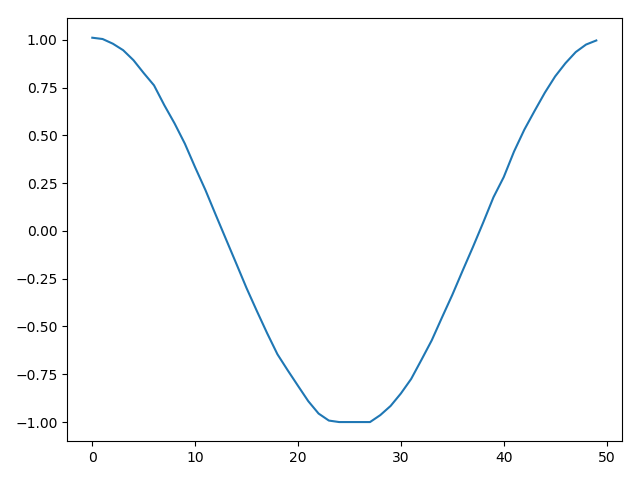另一种方式
我们知道多维多步还有另一种方法，前者是一步一步去填满这个数组，而现在的方法是一维一维去填满这个数组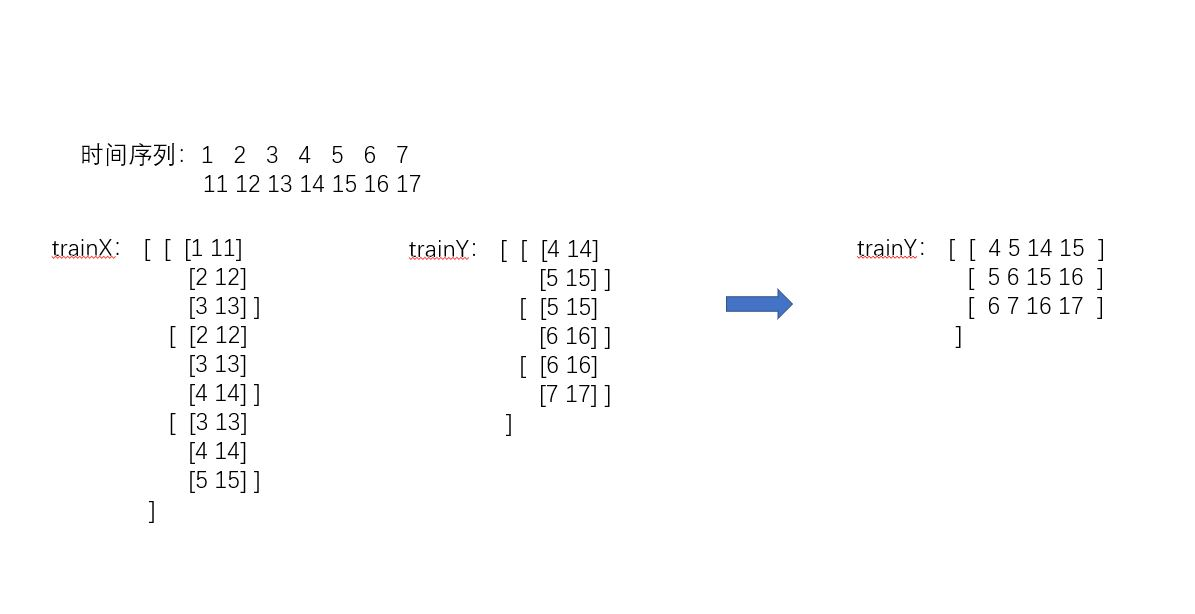转化函数
def create_dataset(data,n_predictions,n_next):
'''
对数据进行处理
'''
dim = data.shape
train_X, train_Y = [], []
for i in range(data.shape-n_predictions-n_next-1):
a = data[i:(i+n_predictions),:]
train_X.append(a)
tempb = data[(i+n_predictions):(i+n_predictions+n_next),:]
b = []
for j in range(dim):
for k in range(len(tempb)):
b.append(tempb[k,j])
train_Y.append(b)
train_X = np.array(train_X,dtype='float64')
train_Y = np.array(train_Y,dtype='float64')

return train_X, train_Y

反转化函数
def reshape_y_hat(y_hat,dim):
re_y = np.zeros(len(y_hat),dtype='float64')
length =int(len(y_hat)/dim)
re_y = re_y.reshape(length,dim)

for curdim in range(dim):
for i in range(length):
re_y[i,curdim] = y_hat[i + curdim*length]

return  re_y

同样的进行刚才的训练，预测结果为：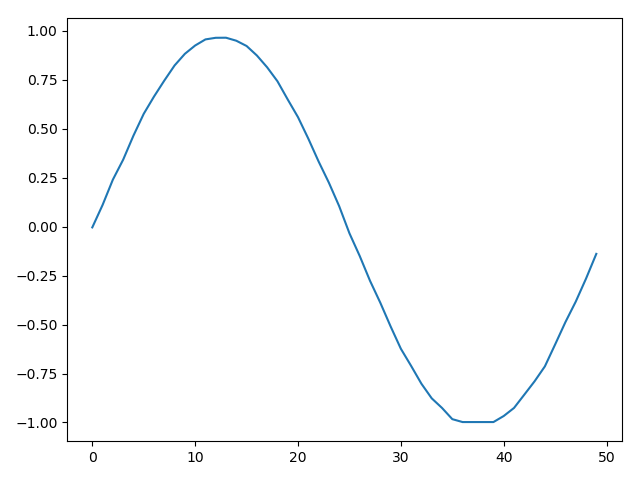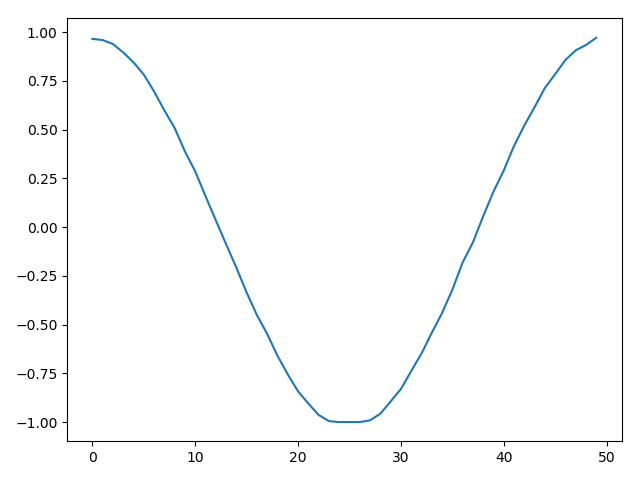总结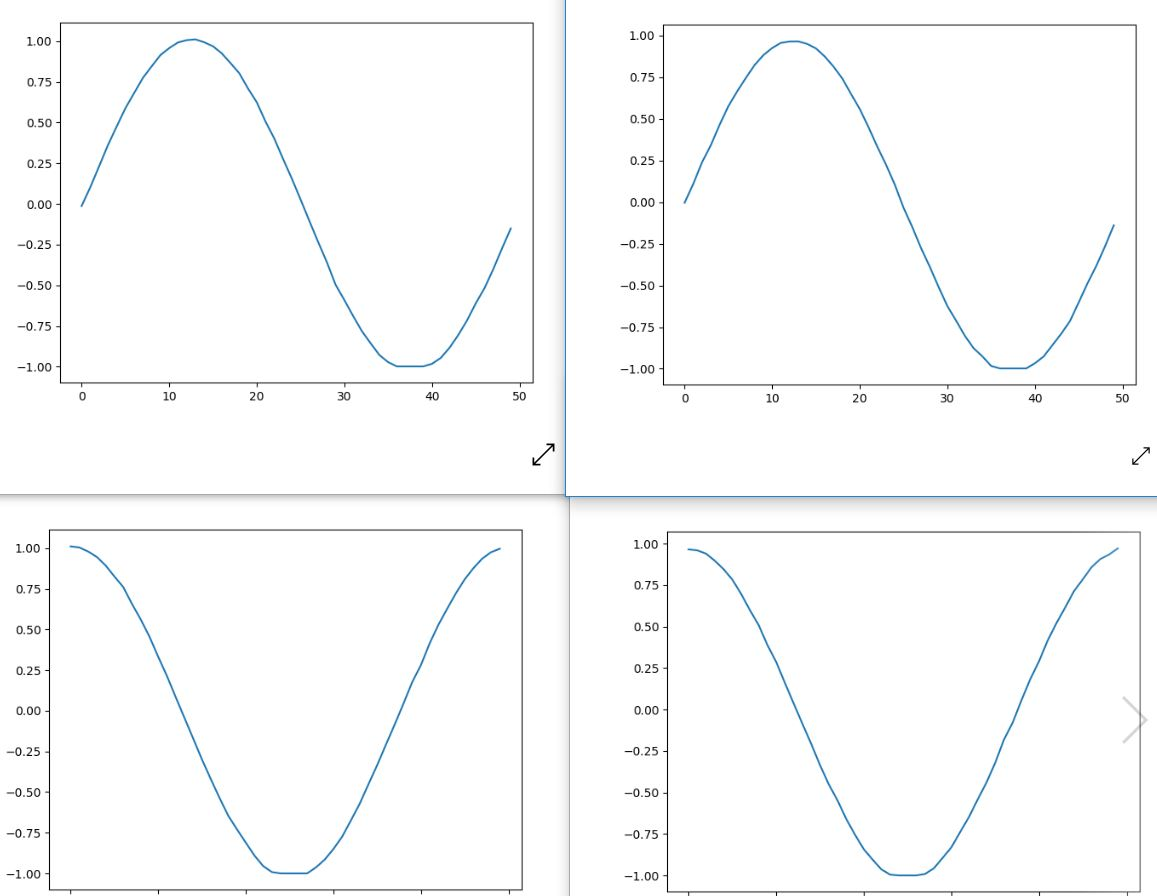左1右2 由图可见两种方法都是可以适用的，但对于维度很大的精确度还带商榷。
同时训练时建议训练数据的步数至少是1-2个周期，预测步数是训练步数的一半-四分之一乃至更少。

注：代码已上传到我的github


展开全文时间序列
• 文章目录一、背景二、主要技术介绍1、RNN模型2、LSTM模型3、控制门工作原理四、代码实现五、案例分析六、参数设置七、结论 一、背景 近年来，股票预测还处于一个很热门的阶段，因为股票市场的波动十分巨大，随时...
文章目录一、背景二、主要技术介绍1、RNN模型2、LSTM模型3、控制门工作原理四、代码实现五、案例分析六、参数设置七、结论完整程序下载
一、背景
近年来，股票预测还处于一个很热门的阶段，因为股票市场的波动十分巨大，随时可能因为一些新的政策或者其他原因，进行大幅度的波动，导致自然人股民很难对股票进行投资盈利。因此本文想利用现有的模型与算法，对股票价格进行预测，从而使自然人股民可以自己对股票进行预测。
理论上，股票价格是可以预测的，但是影响股票价格的因素有很多，而且目前为止，它们对股票的影响还不能清晰定义。这是因为股票预测是高度非线性的，这就要预测模型要能够处理非线性问题，并且，股票具有时间序列的特性，因此适合用循环神经网络，对股票进行预测。
虽然循环神经网络（RNN），允许信息的持久化，然而，一般的RNN模型对具备长记忆性的时间序列数据刻画能力较弱，在时间序列过长的时候，因为存在梯度消散和梯度爆炸现象RNN训练变得非常困难。Hochreiter 和 Schmidhuber 提出的长短期记忆（ Long Short-Term Memory，LSTM）模型在RNN结构的基础上进行了改造，从而解决了RNN模型无法刻画时间序列长记忆性的问题。
综上所述，深度学习中的LSTM模型能够很好地刻画时间序列的长记忆性。
二、主要技术介绍
1、RNN模型
在传统的RNN（循环神经网络）中，所有的w都是同一个w，经过同一个cell的时候，都会保留输入的记忆，再加上另外一个要预测的输入，所以预测包含了之前所有的记忆加上此次的输入。所有RNN都具有一种重复神经网络模块的链式的形式。在标准的RNN中，这个重复的模块只有一个非常简单的结构，例如一个tanh层。
当权中大于1时，反向传播误差时，误差将会一直放大，导致梯度爆炸；当权中小于1时，误差将会一直缩小，导致梯度消失，进而导致网络权重更新缓慢，无法体现出RNN的长期记忆的效果，使得RNN太过健忘。RNN模型的结构如图：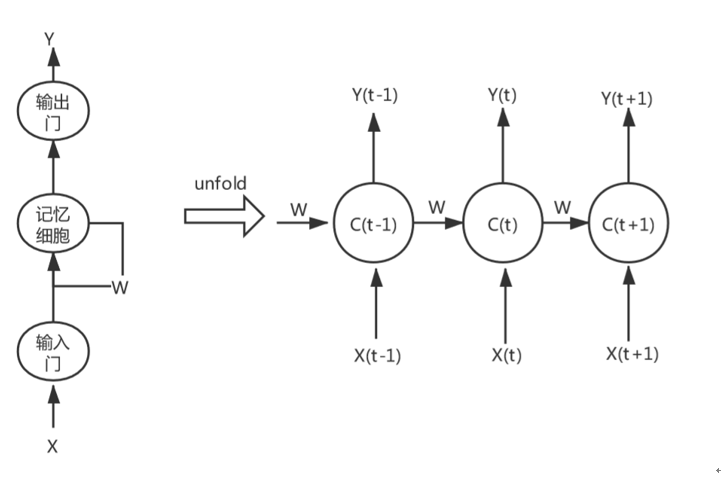2、LSTM模型
长短期记忆模型（long-short term memory）是一种特殊的RNN模型，是为了解决反向传播过程中存在梯度消失和梯度爆炸现象，通过引入门（gate）机制，解决了RNN模型不具备的长记忆性问题，LSTM模型的结构如图：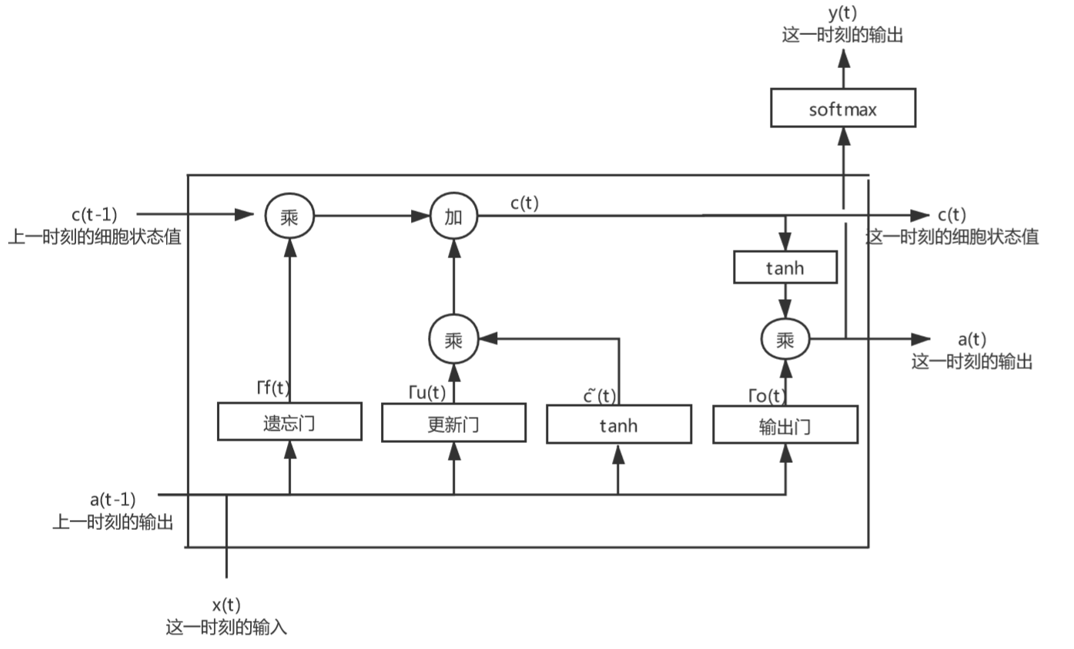具体来说，LSTM模型的1个神经元包含了1个细胞状态（cell）和3个门（gate）机制。细胞状态（cell）是LSTM模型的关键所在，类似于存储器，是模型的记忆空间。细胞状态随着时间而变化，记录的信息由门机制决定和更新。门机制是让信息选择式通过的方法，通过sigmoid函数和点乘操作实现。sigmoid取值介于0～1之间，乘即点乘则决定了传送的信息量（每个部分有多少量可以通过），当sigmoid取0时表示舍弃信息，取1时表示完全传输(即完全记住)。
LSTM 拥有三个门，来保护和控制细胞状态：遗忘门（forget gate）、更新门（update gate）和输出门（output gate）。
细胞状态类似于传送带。直接在整个链上运行，只有一些少量的线性交互。信息在上面流传保持不变会很容易。
如图：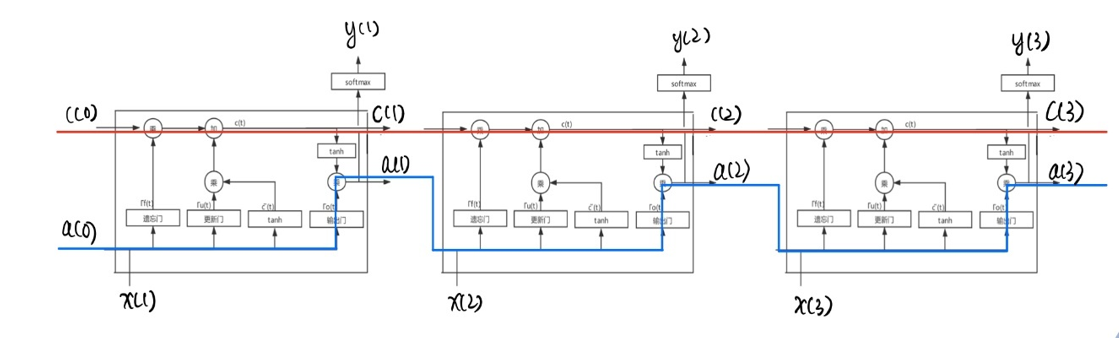3、控制门工作原理
遗忘门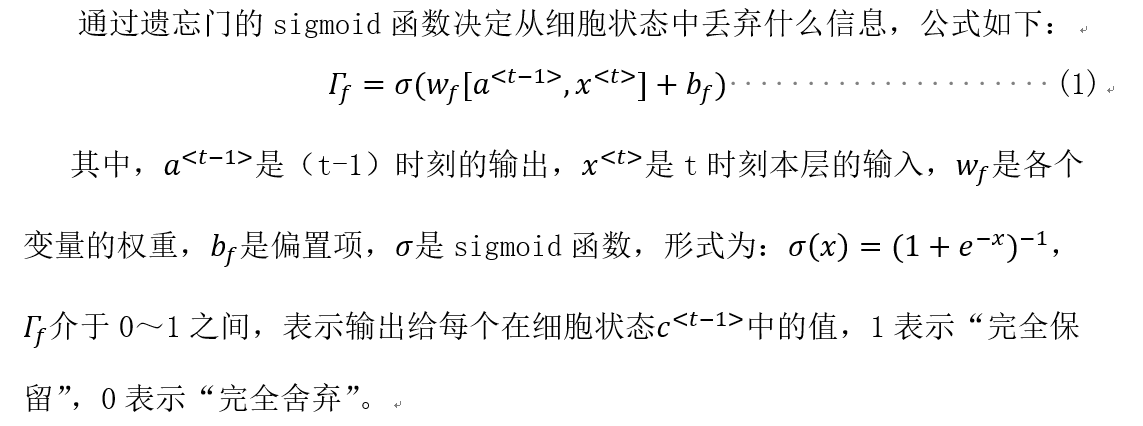更新门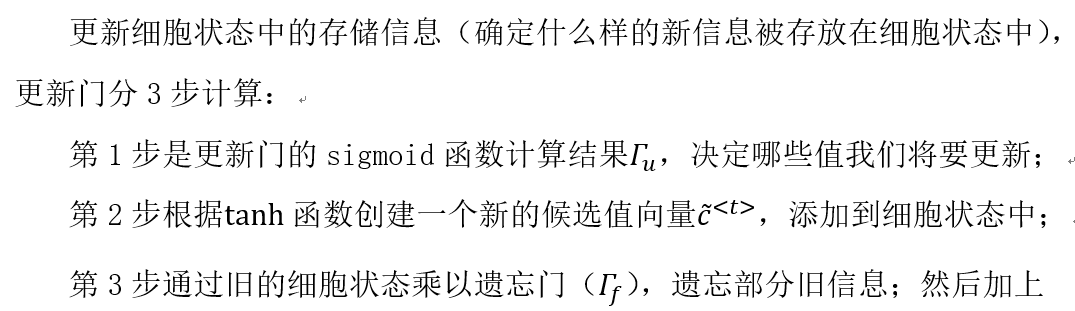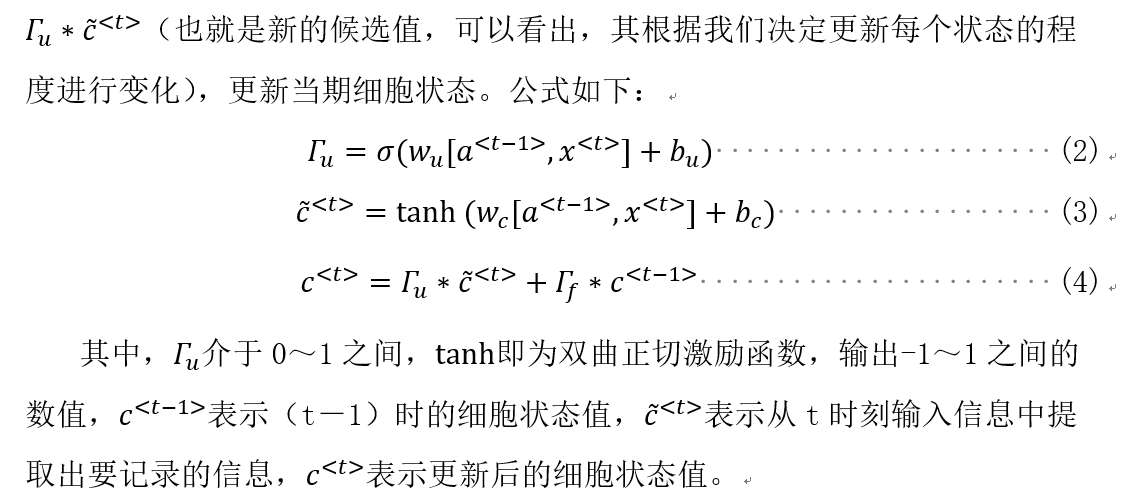输出门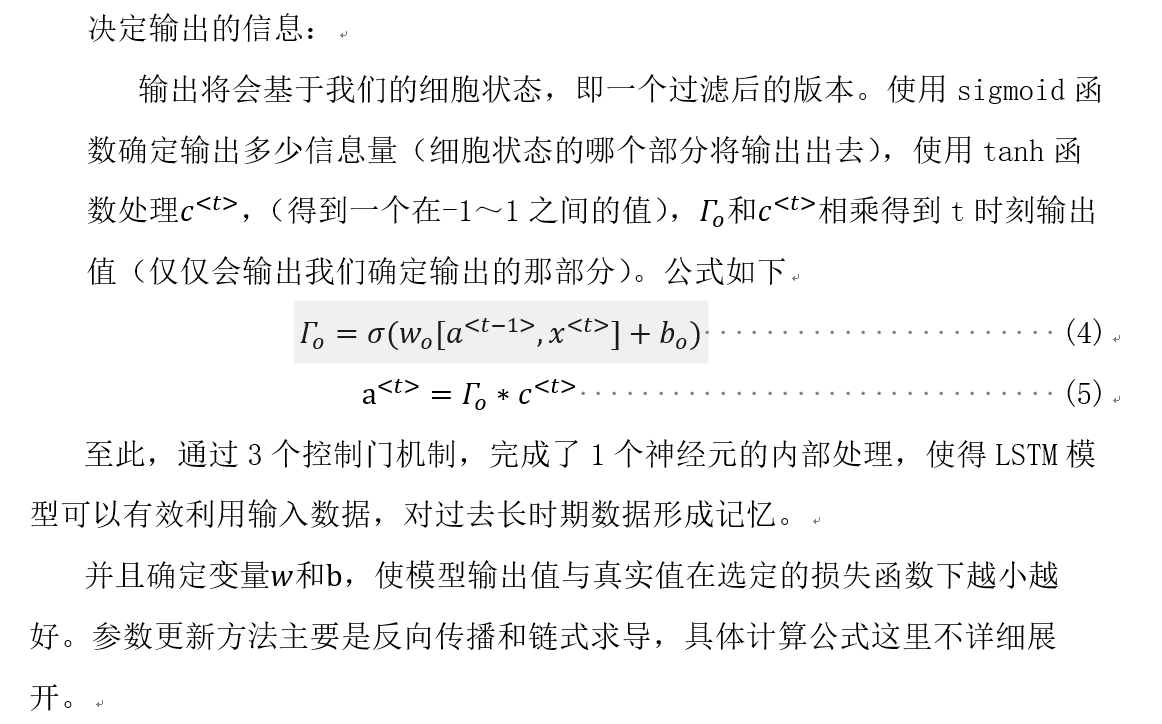四、代码实现
UI
demo.py
import tensorflow as tf
import numpy as np
import tkinter as tk
from tkinter import filedialog
import time
import pandas as pd

import stock_predict as pred

def creat_windows():
win = tk.Tk()  # 创建窗口
sw = win.winfo_screenwidth()
sh = win.winfo_screenheight()
ww, wh = 800, 450
x, y = (sw - ww) / 2, (sh - wh) / 2
win.geometry("%dx%d+%d+%d" % (ww, wh, x, y - 40))  # 居中放置窗口

win.title('LSTM股票预测')  # 窗口命名

f_open =open('dataset_2.csv')
canvas = tk.Label(win)
canvas.pack()

var = tk.StringVar()  # 创建变量文字
var.set('选择数据集')
tk.Label(win, textvariable=var, bg='#C1FFC1', font=('宋体', 21), width=20, height=2).pack()

tk.Button(win, text='选择数据集', width=20, height=2, bg='#FF8C00', command=lambda: getdata(var, canvas),
font=('圆体', 10)).pack()

canvas = tk.Label(win)
L1 = tk.Label(win, text="选择你需要的 列(请用空格隔开，从0开始）")
L1.pack()
E1 = tk.Entry(win, bd=5)
E1.pack()
button1 = tk.Button(win, text="提交", command=lambda: getLable(E1))
button1.pack()
canvas.pack()
win.mainloop()

def getLable(E1):
string = E1.get()
print(string)
gettraindata(string)

def getdata(var, canvas):
global file_path
var.set("注，最后一个为label")
# 读取文件第一行标签
with open(file_path, 'r', encoding='gb2312') as f:
# with open(file_path, 'r', encoding='utf-8') as f:
data2 = lines
print()

canvas.configure(text=data2)
canvas.text = data2

def gettraindata(string):
f_open = open(file_path)
list = string.split()
print(list)
x = len(list)
index=[]
# data = df.iloc[:, [1,2,3]].values  # 取第3-10列 （2:10从2开始到9）
for i in range(x):
q = int(list[i])
index.append(q)
global data
data = df.iloc[:, index].values
print(data)
main(data)

def main(data):
pred.LSTMtest(data)

if __name__ == "__main__":
creat_windows()

stock_predict.py
import numpy as np
import matplotlib.pyplot as plt
import tensorflow as tf
import pandas as pd
import math

def LSTMtest(data):

n1 = len(data) - 1 #因为最后一位为label
n2 = len(data)
print(n1, n2)

# 设置常量
input_size = n1  # 输入神经元个数
rnn_unit = 10    # LSTM单元(一层神经网络)中的中神经元的个数
lstm_layers = 7  # LSTM单元个数
output_size = 1  # 输出神经元个数（预测值）
lr = 0.0006      # 学习率

train_end_index = math.floor(n2*0.9)  # 向下取整
print('train_end_index', train_end_index)
# 前90%数据作为训练集，后10%作为测试集
# 获取训练集
# time_step 时间步，batch_size 每一批次训练多少个样例
def get_train_data(batch_size=60, time_step=20, train_begin=0, train_end=train_end_index):
batch_index = []
data_train = data[train_begin:train_end]
normalized_train_data = (data_train - np.mean(data_train, axis=0)) / np.std(data_train, axis=0)  # 标准化
train_x, train_y = [], []  # 训练集
for i in range(len(normalized_train_data) - time_step):
if i % batch_size == 0:
# 开始位置
batch_index.append(i)
# 一次取time_step行数据
# x存储输入维度（不包括label） :X(最后一个不取）
# 标准化(归一化）
x = normalized_train_data[i:i + time_step, :n1]
# y存储label
y = normalized_train_data[i:i + time_step, n1, np.newaxis]
# np.newaxis分别是在行或列上增加维度
train_x.append(x.tolist())
train_y.append(y.tolist())
# 结束位置
batch_index.append((len(normalized_train_data) - time_step))
print('batch_index', batch_index)
# print('train_x', train_x)
# print('train_y', train_y)
return batch_index, train_x, train_y

# 获取测试集
def get_test_data(time_step=20, test_begin=train_end_index+1):
data_test = data[test_begin:]
mean = np.mean(data_test, axis=0)
std = np.std(data_test, axis=0)  # 矩阵标准差
# 标准化(归一化）
normalized_test_data = (data_test - np.mean(data_test, axis=0)) / np.std(data_test, axis=0)
# " // "表示整数除法。有size个sample
test_size = (len(normalized_test_data) + time_step - 1) // time_step
print('test_size', test_size)
test_x, test_y = [], []
for i in range(test_size - 1):
x = normalized_test_data[i * time_step:(i + 1) * time_step, :n1]
y = normalized_test_data[i * time_step:(i + 1) * time_step, n1]
test_x.append(x.tolist())
test_y.extend(y)
test_x.append((normalized_test_data[(i + 1) * time_step:, :n1]).tolist())
test_y.extend((normalized_test_data[(i + 1) * time_step:, n1]).tolist())
return mean, std, test_x, test_y

# ——————————————————定义神经网络变量——————————————————
# 输入层、输出层权重、偏置、dropout参数
# 随机产生 w,b
weights = {
'in': tf.Variable(tf.random_normal([input_size, rnn_unit])),
'out': tf.Variable(tf.random_normal([rnn_unit, 1]))
}
biases = {
'in': tf.Variable(tf.constant(0.1, shape=[rnn_unit, ])),
'out': tf.Variable(tf.constant(0.1, shape=[1, ]))
}
keep_prob = tf.placeholder(tf.float32, name='keep_prob')  # dropout 防止过拟合

# ——————————————————定义神经网络——————————————————
def lstmCell():
# basicLstm单元
# tf.nn.rnn_cell.BasicLSTMCell(self, num_units, forget_bias=1.0,
# tate_is_tuple=True, activation=None, reuse=None, name=None)
# num_units:int类型，LSTM单元(一层神经网络)中的中神经元的个数，和前馈神经网络中隐含层神经元个数意思相同
# forget_bias:float类型，偏置增加了忘记门。从CudnnLSTM训练的检查点(checkpoin)恢复时，必须手动设置为0.0。
# state_is_tuple:如果为True，则接受和返回的状态是c_state和m_state的2-tuple；如果为False，则他们沿着列轴连接。后一种即将被弃用。
# （LSTM会保留两个state，也就是主线的state(c_state),和分线的state(m_state)，会包含在元组（tuple）里边
# state_is_tuple=True就是判定生成的是否为一个元组）
#   初始化的 c 和 a 都是zero_state 也就是都为list[]的zero，这是参数state_is_tuple的情况下
#   初始state,全部为0，慢慢的累加记忆
# activation:内部状态的激活函数。默认为tanh
# reuse:布尔类型，描述是否在现有范围中重用变量。如果不为True，并且现有范围已经具有给定变量，则会引发错误。
# name:String类型，层的名称。具有相同名称的层将共享权重，但为了避免错误，在这种情况下需要reuse=True.
#

basicLstm = tf.nn.rnn_cell.BasicLSTMCell(rnn_unit, forget_bias=1.0, state_is_tuple=True)
# dropout 未使用
drop = tf.nn.rnn_cell.DropoutWrapper(basicLstm, output_keep_prob=keep_prob)
return basicLstm

def lstm(X):  # 参数：输入网络批次数目
batch_size = tf.shape(X)
time_step = tf.shape(X)
w_in = weights['in']
b_in = biases['in']

# 忘记门（输入门）
# 因为要进行矩阵乘法,所以reshape
# 需要将tensor转成2维进行计算
input = tf.reshape(X, [-1, input_size])
input_rnn = tf.matmul(input, w_in) + b_in
# 将tensor转成3维，计算后的结果作为忘记门的输入
input_rnn = tf.reshape(input_rnn, [-1, time_step, rnn_unit])
print('input_rnn', input_rnn)
# 更新门
# 构建多层的lstm
cell = tf.nn.rnn_cell.MultiRNNCell([lstmCell() for i in range(lstm_layers)])
init_state = cell.zero_state(batch_size, dtype=tf.float32)

# 输出门
w_out = weights['out']
b_out = biases['out']
# output_rnn是最后一层每个step的输出,final_states是每一层的最后那个step的输出
output_rnn, final_states = tf.nn.dynamic_rnn(cell, input_rnn, initial_state=init_state, dtype=tf.float32)
output = tf.reshape(output_rnn, [-1, rnn_unit])
# 输出值，同时作为下一层输入门的输入
pred = tf.matmul(output, w_out) + b_out
return pred, final_states

# ————————————————训练模型————————————————————

def train_lstm(batch_size=60, time_step=20, train_begin=0, train_end=train_end_index):
# 于是就有了tf.placeholder，
# 我们每次可以将 一个minibatch传入到x = tf.placeholder(tf.float32,[None,32])上，
# 下一次传入的x都替换掉上一次传入的x，
# 这样就对于所有传入的minibatch x就只会产生一个op，
# 不会产生其他多余的op，进而减少了graph的开销。

X = tf.placeholder(tf.float32, shape=[None, time_step, input_size])
Y = tf.placeholder(tf.float32, shape=[None, time_step, output_size])
batch_index, train_x, train_y = get_train_data(batch_size, time_step, train_begin, train_end)
# 用tf.variable_scope来定义重复利用,LSTM会经常用到
with tf.variable_scope("sec_lstm"):
pred, state_ = lstm(X) # pred输出值，state_是每一层的最后那个step的输出
print('pred,state_', pred, state_)

# 损失函数
# [-1]——列表从后往前数第一列，即pred为预测值，Y为真实值(Label)
#tf.reduce_mean 函数用于计算张量tensor沿着指定的数轴（tensor的某一维度）上的的平均值
loss = tf.reduce_mean(tf.square(tf.reshape(pred, [-1]) - tf.reshape(Y, [-1])))
# 误差loss反向传播——均方误差损失
# 本质上是带有动量项的RMSprop，它利用梯度的一阶矩估计和二阶矩估计动态调整每个参数的学习率。
saver = tf.train.Saver(tf.global_variables(), max_to_keep=15)

with tf.Session() as sess:
# 初始化
sess.run(tf.global_variables_initializer())
theloss = []
# 迭代次数
for i in range(200):
for step in range(len(batch_index) - 1):
# sess.run(b, feed_dict = replace_dict)
state_, loss_ = sess.run([train_op, loss],
feed_dict={X: train_x[batch_index[step]:batch_index[step + 1]],
Y: train_y[batch_index[step]:batch_index[step + 1]],
keep_prob: 0.5})
#  使用feed_dict完成矩阵乘法 处理多输入
#  feed_dict的作用是给使用placeholder创建出来的tensor赋值

#  [batch_index[step]: batch_index[step + 1]]这个区间的X与Y
#  keep_prob的意思是：留下的神经元的概率，如果keep_prob为0的话， 就是让所有的神经元都失活。
print("Number of iterations:", i, " loss:", loss_)
theloss.append(loss_)
print("model_save: ", saver.save(sess, 'model_save2\\modle.ckpt'))
print("The train has finished")
return theloss

theloss = train_lstm()

# ————————————————预测模型————————————————————
def prediction(time_step=20):

X = tf.placeholder(tf.float32, shape=[None, time_step, input_size])
mean, std, test_x, test_y = get_test_data(time_step)
# 用tf.variable_scope来定义重复利用,LSTM会经常用到
with tf.variable_scope("sec_lstm", reuse=tf.AUTO_REUSE):
pred, state_ = lstm(X)
saver = tf.train.Saver(tf.global_variables())
with tf.Session() as sess:
# 参数恢复（读取已存在模型）
module_file = tf.train.latest_checkpoint('model_save2')
saver.restore(sess, module_file)
test_predict = []
for step in range(len(test_x) - 1):
predict = sess.run(pred, feed_dict={X: [test_x[step]], keep_prob: 1})
predict = predict.reshape((-1))
test_predict.extend(predict)  # 把predict的内容添加到列表

# 相对误差=（测量值-计算值）/计算值×100%
test_y = np.array(test_y) * std[n1] + mean[n1]
test_predict = np.array(test_predict) * std[n1] + mean[n1]
acc = np.average(np.abs(test_predict - test_y[:len(test_predict)]) / test_y[:len(test_predict)])
print("预测的相对误差:", acc)

print(theloss)
plt.figure()
plt.plot(list(range(len(theloss))), theloss, color='b', )
plt.xlabel('times', fontsize=14)
plt.ylabel('loss valuet', fontsize=14)
plt.title('loss-----blue', fontsize=10)
plt.show()
# 以折线图表示预测结果
plt.figure()
plt.plot(list(range(len(test_predict))), test_predict, color='b', )
plt.plot(list(range(len(test_y))), test_y, color='r')
plt.xlabel('time value/day', fontsize=14)
plt.ylabel('close value/point', fontsize=14)
plt.title('predict-----blue,real-----red', fontsize=10)
plt.show()

prediction()

五、案例分析
1、数据说明
本实验分析了两种股票种类，为某单支股票（6109个连续时间点）数据data2上证综合指数前复权日线（6230个连续时间点，1991年到2016年）数据作为data2，分别保存在两个文件中，将两个数据集的最后一列设定为label。前90%数据作为训练集，后10%作为测试集。
Data1: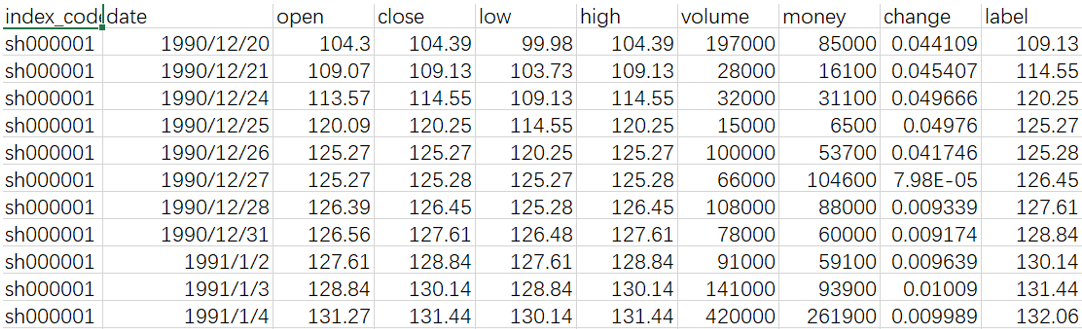Data2: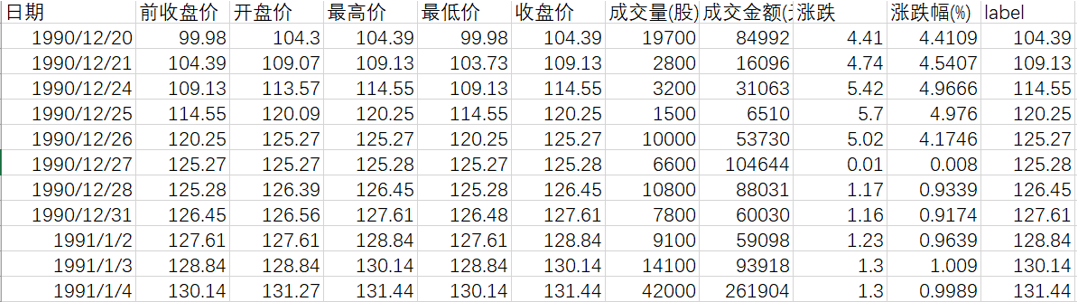本次实验所采用的为LSTM模型：
输入神经元个数 input_size = 选取列数
输出神经元个数 output_size = 1 （预测值个数）
学习率 lr = 0.0006
随机初始化初始化网络权重
2、数据预处理
零-均值规范化（z-score标准化）：
标准化值，是讲集合中单个数与集合的均值相减的结果除以集合的标准差得到的标准化的结果，该方法类似与正态分布标准化转换，转换函数公式为：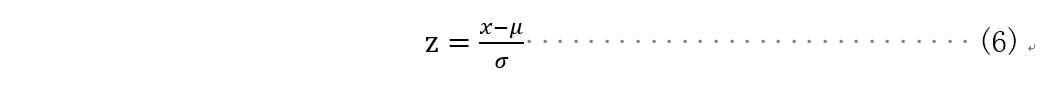公式中x为需要被标准化的原始值，μ为均值，σ为标准差，σ不等于0。
Z分数标准化处理后的值代表原始值和集合均值之间的举例，以标准差为单位计算。该值存在正负值，低于均值均为辅助，反之则为证书，其范围为[-∞，+∞],数据均值为0，方差为1。
3、损失函数
损失函数（Loss function）是用来估量网络模型的预测值X与真实值Y的不一致程度，它是一个非负实值函数，通常用 L(Y,f(x))来表示。损失函数越小，模型的鲁棒性就越好。损失函数是经验风险函数的核心部分，也是结构风险函数的重要组成部分。
本实验采取十分常用的均方误差损失：
平方损失也可以理解为是最小二乘法，一般在回归问题中比较常见，最小二乘法的基本原理是：最优拟合直线是使各点到回归直线的距离和最小的直线，即平方和最。同时在实际应用中，均方误差也经常被用为衡量模型的标准：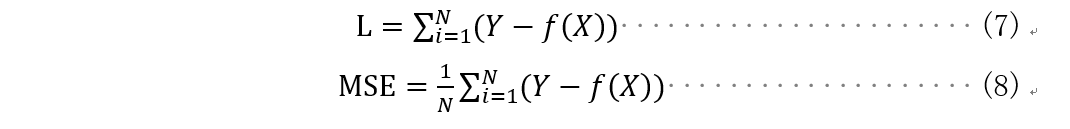4、误差标准
相对偏差是指某一次测量的绝对偏差占平均值的百分比。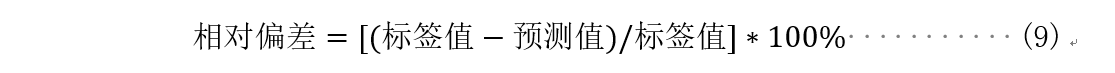5、可视化UI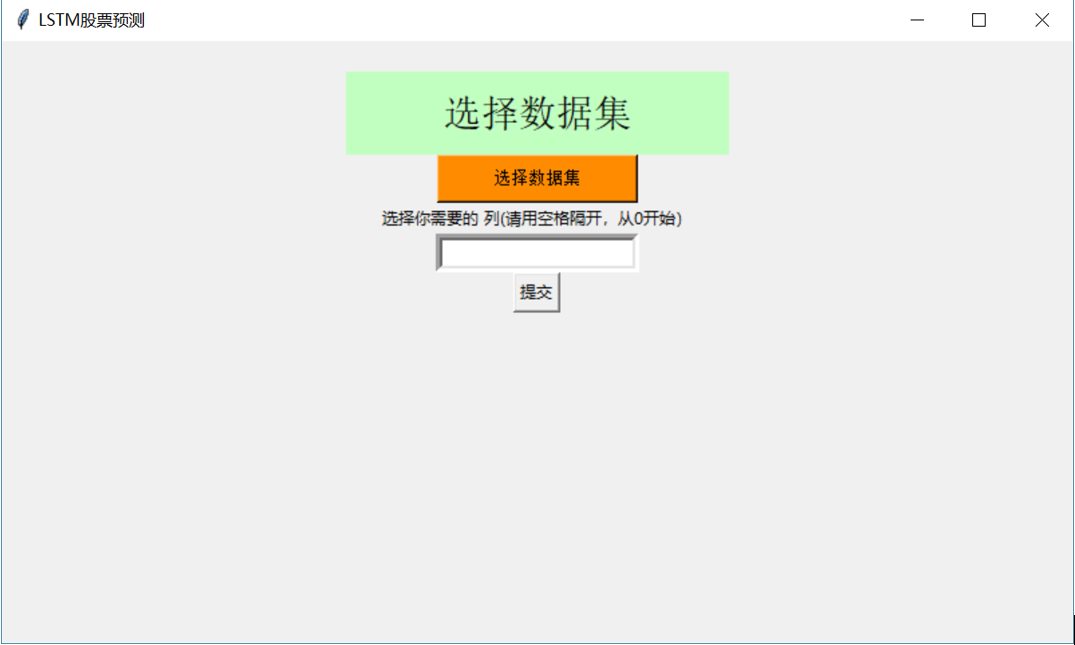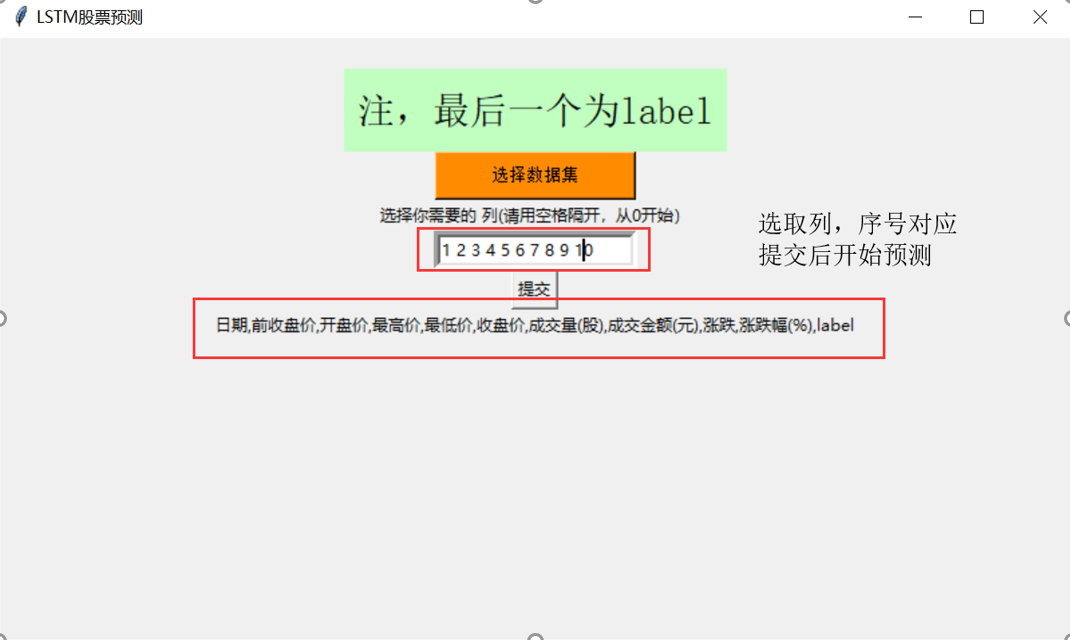六、参数设置
1、输入维度及迭代次数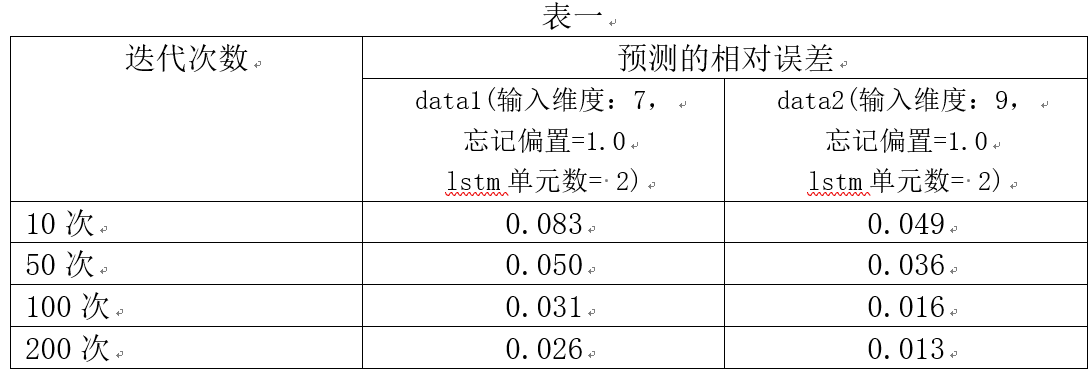由表一可见，输入维度越多，网络训练效果越好；迭代次数在100次时，网络已经比较稳定。
2、忘记偏置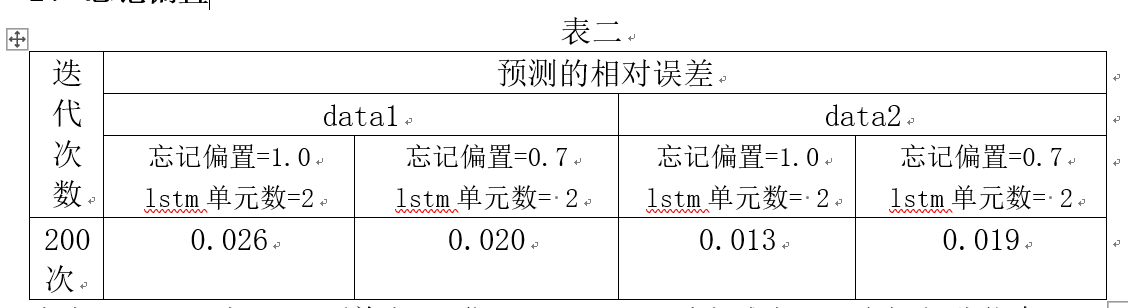由表二可见，在data1(单支股票)forget_bias适当减小，即忘记部分信息，网络训练效果有些许提高，但在data2(大盘)中，网络训练效果却有所下滑。个人认为，可能是因为对于单支股票来说，近2天的数据相关程度比较小，而在大盘中，因为近2天的数据相关程度比较大，毕竟有多方面因素影响股价。
3、LSTM单元数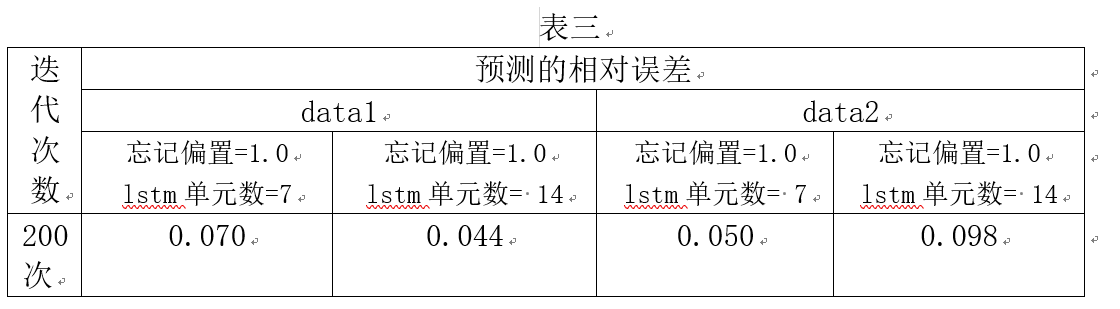由表三可见，两个数据集中，LSTM单元数增加的情况下时，网络训练效果反而下降，可以看出，其实股票行情在7天内的的相关联程度比在14天内的情况高，但是有可能是因为forget_bias过大。因此，在进行一组实验，调整forget_bias值进行比较。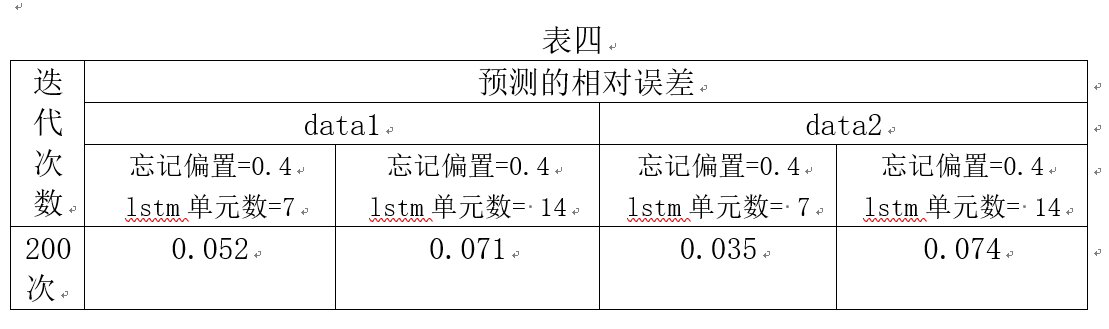由表四可以看出，在相同LSTM单元数的情况下，forget_bias较小时，预测效果较好，我们可以看出，在LSTM单元数较大的情况下，forget_bias应选取比较小的，以免记忆太多无效信息。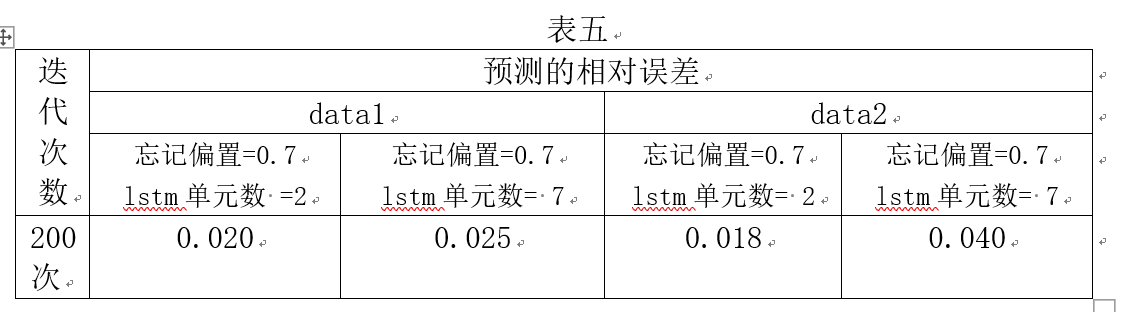由表五可以看出，在data1和data2两个数据集中，LSTM单元数较小的情况下，forget_bias比较大时，预测效果较好，记忆更多相关信息。因此LSTM单元数较小的情况下，forget_bias应选取比较大的，记忆更多相关信息。
4、可视化结果
选取数据集data1，迭代次数为200次
（1）、忘记偏置=1.0 ， LSTM单元数 = 2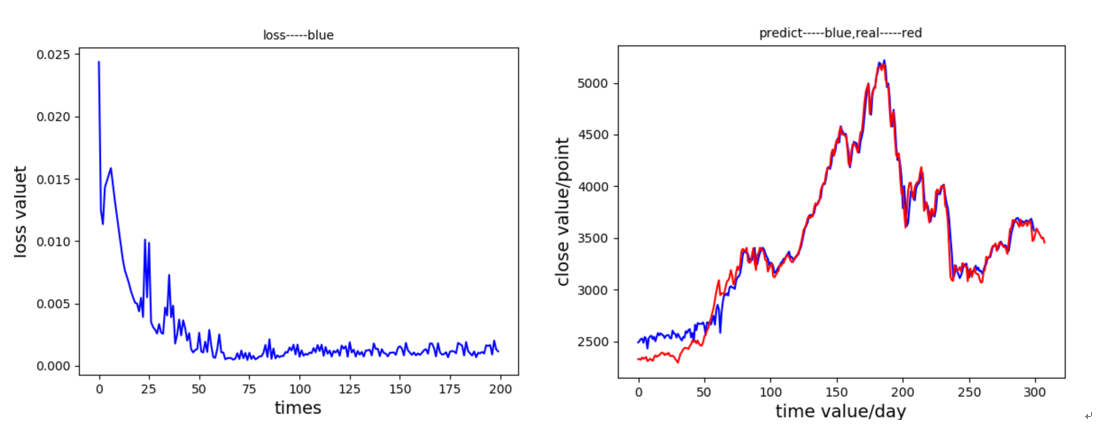（2）、忘记偏置=0.7 ， LSTM单元数 = 2（表现最好）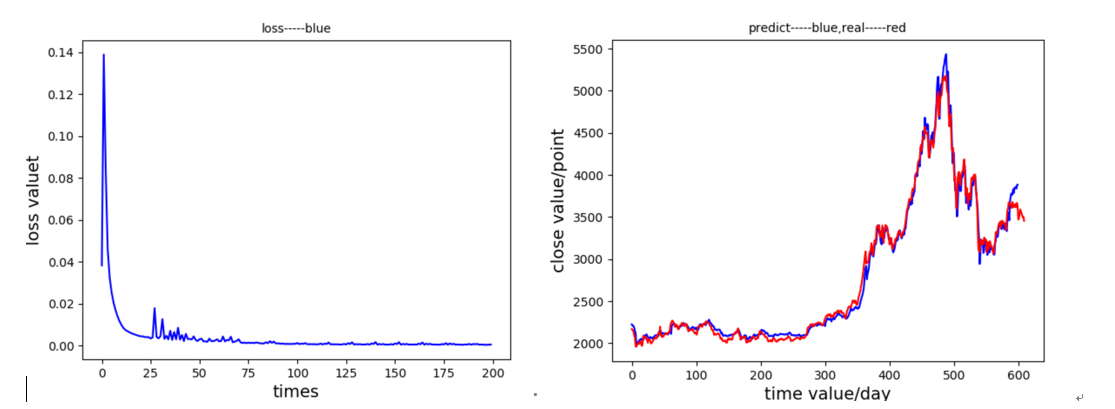（3）、忘记偏置=1.0 ， LSTM单元数 = 7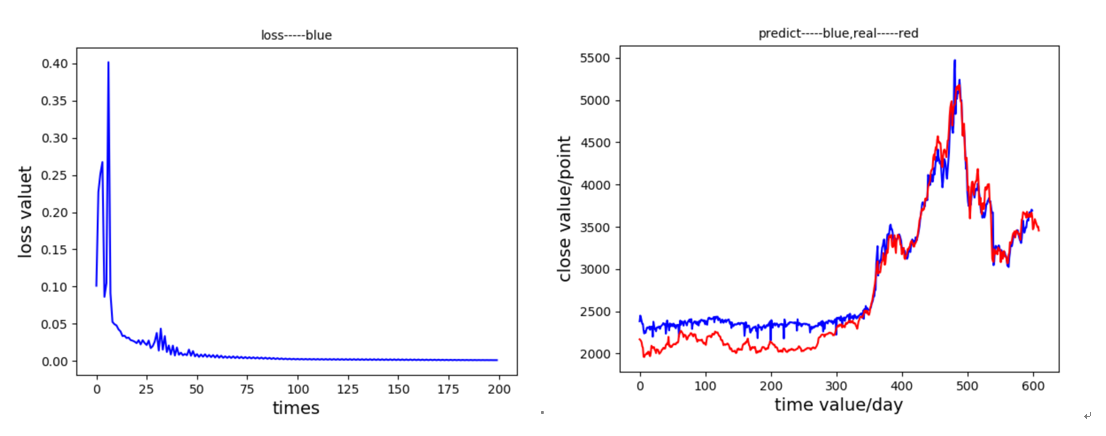（4）、忘记偏置=1.0 ， LSTM单元数 = 14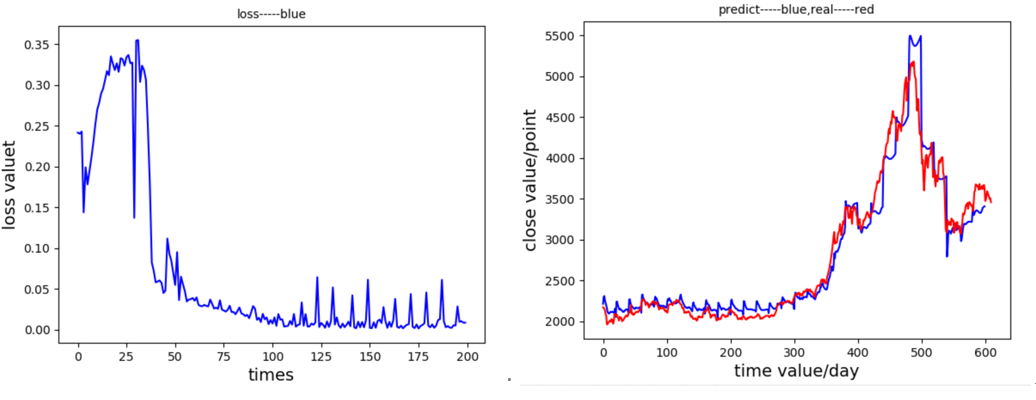（5）、忘记偏置=0.7， LSTM单元数 = 7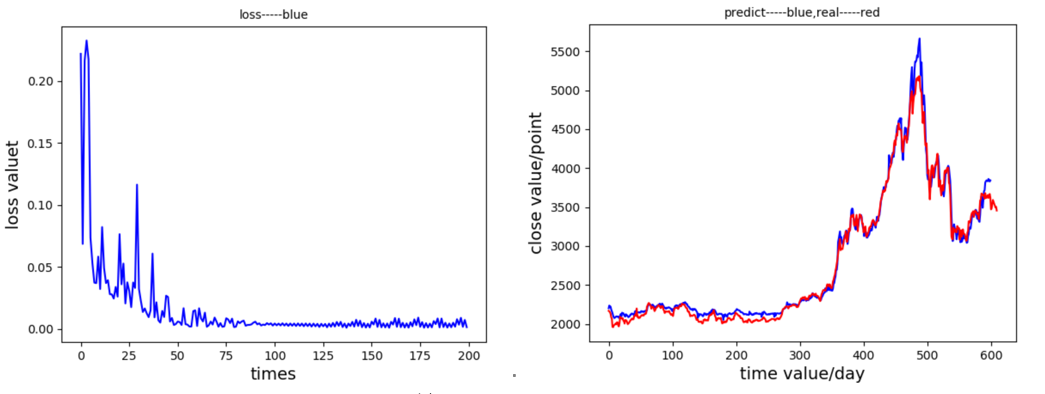（6）、忘记偏置=0.4 ， LSTM单元数 = 7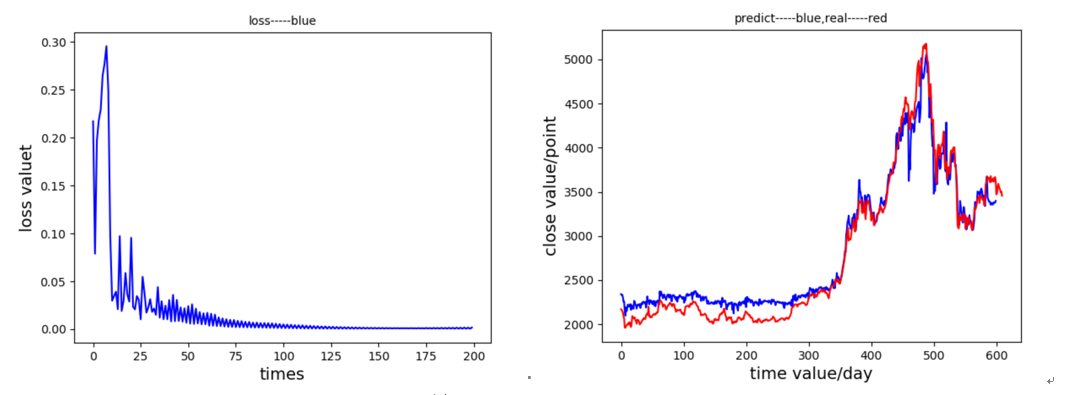（7）、忘记偏置=0.4 ， LSTM单元数 = 14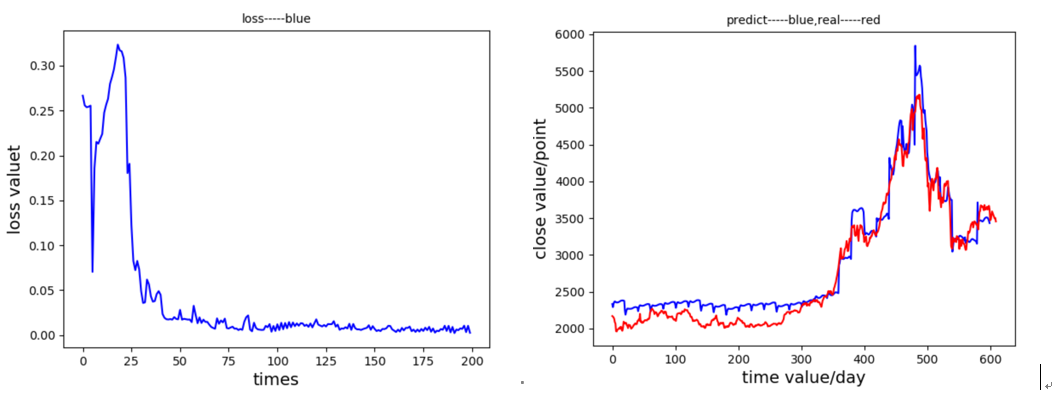七、结论
针对以上实验，可以得知，在LSTM模型下的对股票收盘价预测值较为准确和稳定。对LSTM模型进行参数调整，发现迭代次数在100次后，网络模型趋于稳定，说明其是一个较轻量级的网络；在LSTM单元数较大的情况下，forget_bias应选取比较小的，以免记忆太多无效信息；LSTM单元数较小的情况下，forget_bias应选取比较大的，记忆更多相关信息。当然，这和本身的数据集有关。就股票数据集来说，本实验中表现的最优秀的是，忘记偏置为0.7，LSTM神经单元数取2时，网络预测效果最好，说明，在2天内股票序列是比较有价值的，与最后预测值有一定程度的联系。
完整程序下载
自行调试运行噢~
参考文献
 陈卫华. 基于深度学习的上证综指波动率预测效果比较研究[D].统计与信息论坛，2018.
 Hochreiter & Schmidhuber. Long short-term memory［ J］. Neural Computation， 1997， 9（ 8）.
参考博客
https://blog.csdn.net/jiaoyangwm/article/details/79725445
https://blog.csdn.net/mylove0414/article/details/56969181
本文为原创。
转载请注明出处。


展开全文时间序列 python实现
• 短期电力负荷预测是电力系统安全调度、...本文将LSTM用于短期电力负荷预测 , 提出基于LSSVM 的短期电力负荷预测模型 , 同时建立改进粒子群模型对 LSTM进行参数优化 , 并以浙江某地区的历史负荷数据和气象数据为例进...
采用tensorflow1.x编写，python

短期电力负荷预测是电力系统安全调度、经济运行的重要依据 , 随着电力系统的市场化 , 负荷预测的精度直接影响到电力系统运行的可靠性、经济性和供电质量。LSTM 为短期电力负荷预测提供了一个新的研究方向。本文将LSTM用于短期电力负荷预测 , 提出基于LSSVM 的短期电力负荷预测模型 , 同时建立改进粒子群模型对 LSTM进行参数优化 , 并以浙江某地区的历史负荷数据和气象数据为例进行验证 , 实例验证表明 , 改进 PSO-LSTM 模型的预测效果明显提高。

1,改进的PSO算法，提出对PSO的惯性权重进行改进，改进后对5维sphere函数的极值寻优结果如图所示: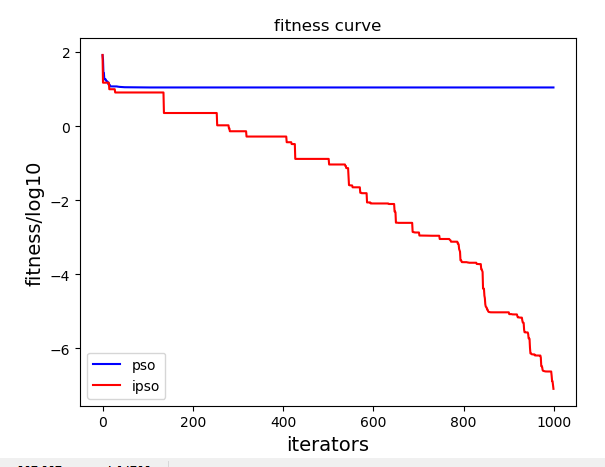如图所示为PSO与IPSO对5维sphere函数极值寻优，从图上可以清晰看出，PSO算法大约在60次迭代时就早早的陷入了局部最优解，最终值约为1；IPSO极大的增强了寻优能力，在1000次迭代时依然具有寻找更优解的能力，极值大约为10^-7次方，这与PSO的结果相差6个数量级，明显可以IPSO拥有更强的寻优能力。

2, 基于LSTM的短期电力负荷预测。

本文选择的是浙江某地区的电力负荷值，每天的数据包括平均温度、最高温度、最低温度、相对湿度、星期类型、与24个时刻的负荷，共29个特征。基于LSTM，本文选择以第n-1天的29个值与第n天的平均温度、最高温度、最低温度、相对湿度、星期类型作为输入，以第n天的24个时刻的负荷作为输出，构建34输入24输出的LSTM短期电力负荷预测模型。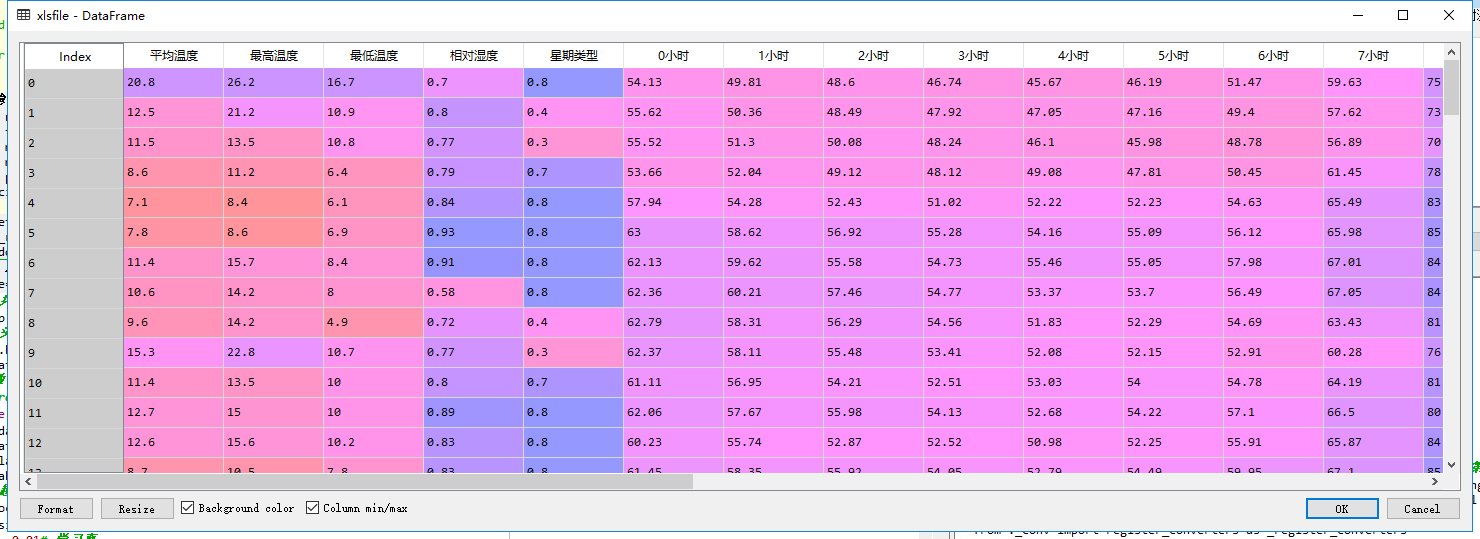数据集一共96个样本，选择95个样本作为训练集，剩下1个样本作为测试集，相当于预测最后一天得到的结果如图所示。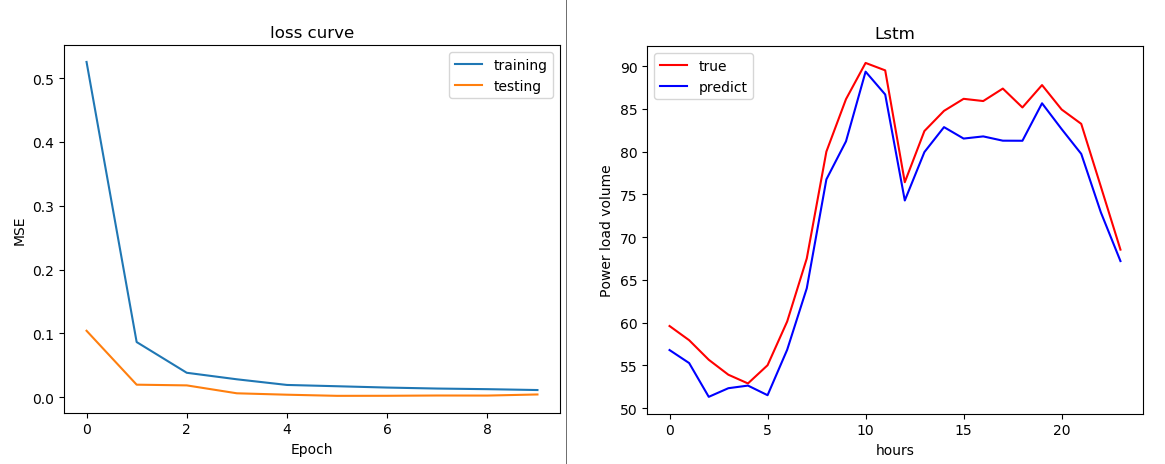LSTM测试集的mape: 0.04030398741922454  rmse: 3.254871212868774  mad: 2.982307053878904  R2: 0.9385667729662959  tic: 0.021831448577835744，可见lstm的泛化能力较好。此外，由于LSTM参数众多，本文拟采用上述改进PSO算法对LSTM的超参数进行寻优，寻优参数包括迭代次数、学习率、隐含层神经元节点数。

3，基于IPSO-LSTM的短期电力负荷预测

基于IPSO的LSTM短期电力负荷预测，以最小化0.5*(测试集mape+训练集mape）作为适应度函数。适应度曲线与各超参数的变化曲线如下图所示。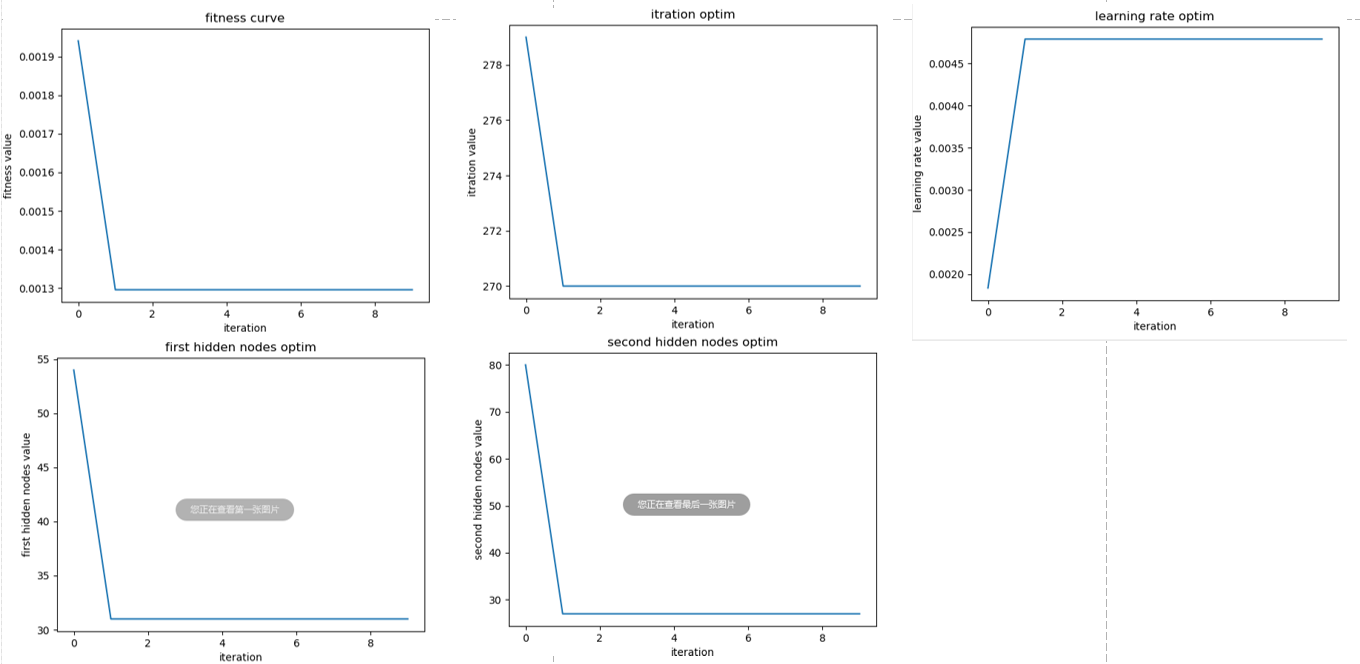对比结果如下，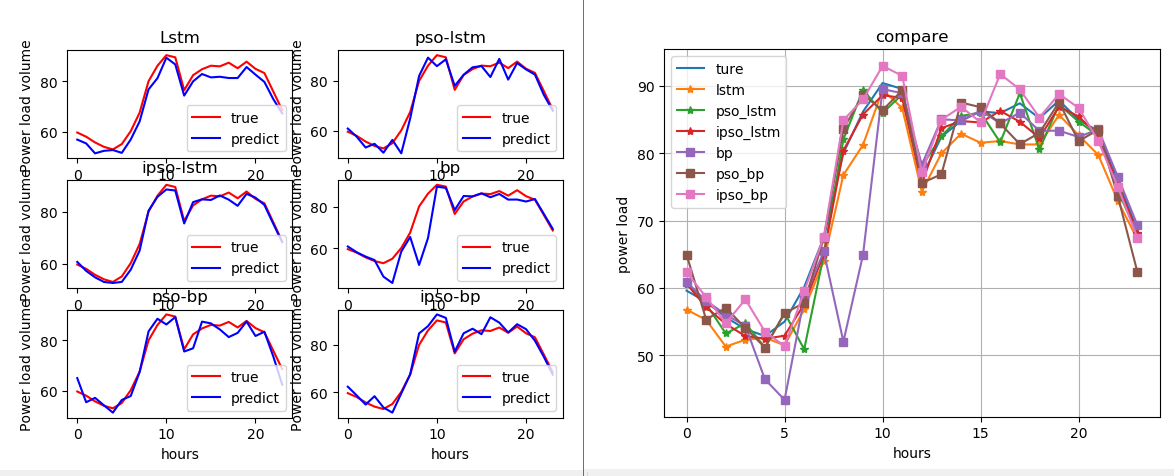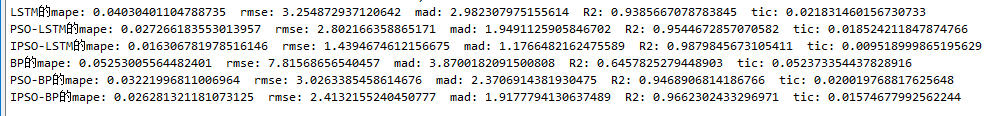上述结果说明，ipso-lstm确实能在一定程度减小误差；第二，lstm本身泛化能力也不错，即使不用优化也能达到一个不错的结果，经过分析，这与很多因素有关，比如我们的数据较为简单。https://github.com/fish-kong/PSO-IPSO-LSTM-regression

展开全文• ## LSTM股票预测

千次阅读 2018-11-21 18:08:04
LSTM股票预测 LSTM股票预测 使用开盘价、最高价、最低价、收盘价、交易量预测下一个交易日的收盘价。 模型还不完善，欢迎交流。 import tensorflow as tf import numpy as np import matplotlib.pyplot as plt ...
• 源程序代码 详解 股票预测 LSTM 时间序列rnn 代码程序数据集下载压缩包
• 分解处理，之后基于LSTM模型来实现时序数据的建模预测分析。 对于现在的我来说，属于数据信号处理领域里面的小白，所以写这篇文章很可能会有错误或者是不合理的地方，如果问题欢迎指出，欢迎交流学习，同时呢？...
• ## LSTM算法+数据预测

万次阅读 热门讨论 2018-11-06 20:43:20
传统的神经网络一般都是全连接结构，且非相邻两层之间是没有连接的。对输入为时序的样本无法解决...因此引入LSTM 1 LSTM算法小结  LSTM：是对RNN算法的改进，通过不同三个门控制之前信息对当前信息的影响即（1：那...
• 接下来来介绍一下关于灰色预测模型的基本建模过程： 1、打开MATLAB软件，在其主界面的编辑器中写入下列程序： function []=greymodel(y) % 本程序主要用来计算根据灰色理论建立的模型的预测值。 % 应用的数学模型...
• PM2.5数据LSTM实现预测数据集说明主要步骤环境全部代码转化为监督数据导入数据集归一化转化为监督数据删除不需要预测的列分割为训练集和数据LSTM反归一化真实值与预测值的对比 数据集说明 本实验采用的PM2.5空气...tensorflow 机器学习 深度学习 神经网络
• 对于较为简单的时间序列预测问题，可以使用Exponential Smoothing...然而，对于复杂的时间序列预测问题，LSTM不失为一种很好的选择。因此，本文旨在探讨如何利用LSTM神经网络求解时间序列预测问题。首先，需要明白时...Keras
• 基于Keras的LSTM多变量时间序列预测 https://blog.csdn.net/sinat_22510827/article/details/80996937
• 使用Keras LSTM预测时间序列 参考文章： Kesci： Keras 实现 LSTM——时间序列预测：https://www.cnblogs.com/mtcnn/p/9411597.html 读取数据 data_path = "/mnt/X500/farmers/tongyao/机器学习项目练习/industry_...
• 深度学习 股票预测代码lstm+数据.rar 深度学习 股票预测代码lstm+数据.rar
• 训练好LSTM模型（单变量）后，现在需要进行数据预测。请教下该怎么做？ 早期的LSTM模型训练时，数据是每隔4小时为一个数据，time-step设置为7. 现在要预测未来7天的数据。有点不清楚具体该如何处理了。。求指点。...python 人工智能 机器学习 深度学习 神经网络
• 数据科学学习之旅中，我经常处理日常工作中的时间序列数据集，并据此做出预测Python xgboost 时间序列
• 1. project的文件基本结构 2. project配置文件config.py path_to_dataset = '../my_data/international-airline-passengers.csv' split_rate = 0.9 infer_seq_length = 10 # 用于推断的历史序列长度 ...
• 网上的教程多是用正余弦数据在做预测，输入输出都是一维，我这用波士顿房价，输入是13个特征！注意与前面两个模型不同的是，没有用train_test_split把训练数据分割，而是用的时序数据。代码中注释比较少，不明白的...
• 时隔半年多，毕设男孩终于重操旧业，回到了 LSTM进行时间序列预测和异常检测的路上。 如果有阅读过我之前的博客，可以发现使用 LSTM作单类的时间序列异常检测也是基于对于时间序列的预测进行 登堂入室LSTM：使用LSTM...注意力机制 attention
• 在我之前的文章中，已经对LSTM的实际应用有过很多的实践和说明了，今天介绍的LSTM模型跟之前的不同，在以往的时序数据建模中，我们的输入端是只有一个的，也就是说入口处只有“单条通路”，本文提及的两路LSTM，是在...
• 基于Keras的LSTM多变量时间序列预测 　传统的线性模型难以解决多变量或多输入问题，而神经网络如LSTM则擅长于处理多个变量的问题，该特性使其有助于解决时间序列预测问题。  　 　在接下来的这篇博客中，你将学会...
• DL之LSTM之UvP：基于TF利用LSTM基于DIY时间训练1200个数据预测后200个数据状态 目录 输出结果 设计思路 训练记录全过程 输出结果 设计思路 训练记录全过程 INFO:tensorflow:loss = 0....
• MATLAB实现LSTM多步...时间序列 | 数据预测 | MATLAB】LSTM多步预测 | Elman多步预测 | RNN多步预测 | 机器学习模型 回归预测 | 数据预测 | MATLAB】LSTM多入多出回归预测 | BP多入多出回归预测 | 级联模型预测 ...机器学习 神经网络 深度学习 matlab 人工智能
• 引言这篇博客衔接上一篇博客： Holt-Winters模型原理分析及代码实现（python)，我们在三次指数平滑的基础上，来进一步讨论下对时序数据预测LSTM原理分析(参考博文：Understanding LSTM Networks）Long Short ...
• 使用LSTM神经网络进行时间序列数据预测分析。 基于Tensorflow框架、Kerase接口开发网络模型。 包含数据清洗，数据特征提取，数据建模，数据预测。 想学习更多深度学习项目，可访问如下链接 1.通过自回归(AR,ARIMA)...
• ## LSTM 时间序列预测 matlab

万次阅读 多人点赞 2017-05-02 15:49:28
由于参加了一个小的课题，是关于时间序列预测的。平时习惯用matlab， 网上这种资源就比较少。 借鉴了 http://blog.csdn.net/u010540396/article/details/52797489  的内容，稍微修改了一下程序。 程序说明：DATA....
• 自从LSTM网络提出以来，陆陆续续又出现了很多相关的变种网络，今天从网上找到了一份环境气象领域相关的数据集，可以用于时序数据的建模分析，这里就基于这个数据集来实战双向LSTM网络的时序建模。 这是一张比较...
• DL之LSTM：基于tensorflow框架利用LSTM算法对气温数据集训练并回归预测 目录 输出结果 核心代码 输出结果 数据集 tensorboard可视化 iter: 0 loss: 0.010328549 iter: 500 loss: 0....
• ## GM(1,1)灰色预测模型

千次阅读 2019-10-31 14:30:22
数据分析领域，人们根据数据系统的特点将数据系统分为白色系统，黑色系统和灰色系统。白色系统是说系统内部特征清楚明了，信息完全透明，黑色系统意味着外界对系统内部完全不了解，只能通过外界的联系加以观察研究......